Mathematics
Easy

Question

# Rewrite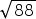into simplest form

##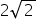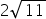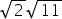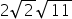Hint:

## The correct answer is:### Here, we have to rewrite √88 in simplest form.Now, √88 = √ ( 2 × 2 × 2 × 11 ) .= √ ( 23 × 11 ) .= √ ( 22 × 2 × 11 ).= 2 √ 2 × 11= 2 √2 √11 .Hence, the correct option is (d).

The square root is an inverse of method of squaring a number.

### Related Questions to study#### With Turito Foundation.#### Get an Expert Advice From Turito.